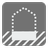Number Base
Simple
ES FR HU IT JA RU UK ZH-HANS English DEDo you remember the radix and Numeral systems from math class? Let's practice with it.

You are given a positive number as a string along with the radix for it. Your function should convert it into decimal form. The radix is less than 37 and greater than 1. The task uses digits and the letters A-Z for the strings.

Watch out for cases when the number cannot be converted. For example: "1A" cannot be converted with radix 9. For these cases your function should return -1.

Input: Two arguments. A number as string and a radix as an integer.

Output: The converted number as an integer.

Example:

```checkio("AF", 16) == 175
checkio("101", 2) == 5
checkio("101", 5) == 26
checkio("Z", 36) == 35
checkio("AB", 10) == -1
```

How it is used: Here you will learn how to work with the various numeral systems and handle exceptions.

Precondition:
re.match("\A[A-Z0-9]\Z", str_number)
0 < len(str_number) ≤ 10